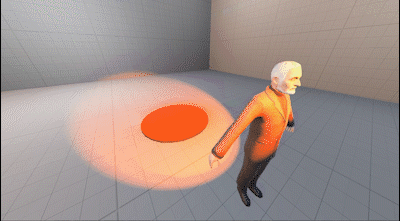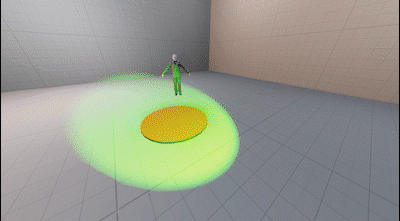# Expressions and Drivers

### Math Expressions

Any animatable actor property (color, radius, position, etc.) can be animated with a math expression (Similar to SFM's expression operators). The implementation is based on the high-performance exprtk library, which includes support for:

• Logical operators (and/or/not/etc.)
• Conditions (if/ternary/etc.)
• Loops (for/while/etc.)
• Vectors
##### Examples

As a basic example, let's say you want to animate the position of your actor, so you move it to [0,30,0] at timestamp 0, [0,50,20] at timestamp 1 and [30,0,-20] at timestamp 2. You can then apply the math expression `value *2` to the property, which will double the values, meaning your actor will move from [0,60,0] to [0,100,40] and then to [60,0,-40] instead.

Here are some other basic examples:

• `var newValue := {0,value,0}; return [newValue];`: Returns [0,value.y,0], which means the actor will only move on the y-axis.
• `var newValue := {time *100,0,0}; return [newValue];`: The channel value will be ignored, and the actor will move on the x-axis depending on how much time has passed since the start of the animation.
• `var f := time /duration; var newValue := {sin(f *pi *2) *100,0,cos(f *pi *2) *100}; return [newValue];` The channel value will be ignored, and the actor will move in a circular motion depending on the time passed.
##### Inputs

These are the variables available for use with math expressions:

• value: The current value
• time: The current time in seconds
• timeIndex: The index into the `times` array of the channel for the current timestamp.
• startOffset: The channel's start offset in seconds.
• timescale: The channel's time scale.
• duration: The duration of the channel (i.e. max time value in the `times` array).

Constants:

• pi
• epsilon
• inf
##### Base Functions
• All built-in exprtk functions
• `float noise(float v1,float v2,float v3)`: Perlin noise.
• `float[X] value_at(float time)`: Returns the value at the specified timestamp. The return value is either `float`, `float[3`] or `float`, depending on the channel value type.
• `float sqr(float v)`: returns v *v
• `float ramp(float x,float a,float b)`: returns (a == b) ? a : (x -a) /(b -a)
• `float cramp(float v1,float v2,float v3)`: returns clamp(ramp(v1,v2,v3),0,1)
• `float lerp(float x,float a,float b)`: returns a +(b -a) *x
• `float clerp(float 1,float v2,float v3)`: returns clamp(lerp(v1,v2,v3),v2,v3)
• `float elerp(float v1,float v2,float v3)`: returns ramp(3 *v1 *v1 -2 *v1 *v1 *v1,v2,v3)
• `float rescale(float x,float xa,float xb,float ya,float yb)`: returns lerp(ramp(x,xa,xb),ya,yb)
• `float crescale(float v1,float v2,float v3,float v4,float v5)`: returns clamp(rescale(v1,v2,v3,v4,v5),v4,v5)
• `print(...)`: For debugging purposes, prints the specified arguments to the console.
##### Quaternion Functions
• `q_from_axis_angle(float axis,float angle,float out)`
• `q_forward(float quat,float out)`
• `q_right(float quat,float out)`
• `q_up(float quat,float out)`
• `q_slerp(float q0,float q1,float factor,float out)`
• `q_lerp(float q0,float q1,float factor,float out)`
• `q_dot(float q0,float q1)`
• `q_mul(float q0,float q1,float out)`
• `q_inverse(float quatInOut)`
• `float q_length(float quat)`

Contrary to SFM's expression operators, PFM's math expressions cannot reference anything outside of the animation channel for the property it's assigned to. To create dependencies between properties, you need to use animation drivers instead.

### Animation Drivers

Animation drivers are similar to math expressions, with a few key differences:

• Lua expression instead of a math expression
• Can be used to create dependencies between arbitrary properties (this is their primary purpose)
• Similar to Blender's driver system
• Slower to compute than math expressions, use sparingly

Since animation drivers are Lua-based, that means you have the entire Lua API available, making them much more powerful tools compared to math expressions. Their purpose is to animate a property programmatically with dependencies to other properties. To do so, animation drivers can have input variables, which can be references to actors, components or component properties.

##### Examples

Animation drivers cannot be created through PFM yet, so the following are Lua-based examples. These may seem a little daunting, but once the system is integrated into the interface, drivers will be much easier to use.

• Changing the color of a light source depending on its distance to an actor (red = actor is close, green = actor is far away):
``````local actorId = "327b5bb8-74ae-400e-bbf7-61a2ad2020d3" -- Id of the actor for whom we check the distance
local expr = [[
local dist = trLight:GetDistance(trActor)
return Color.Red:Lerp(Color.Lime,math.clamp((dist -120) /150.0,0.0,1.0)):ToVector()
]]
ents.COMPONENT_COLOR,"color",
game.ValueDriverDescriptor(expr,{
-- 'trActor' variable referencing the transform component of the actor
["trActor"] = "pragma:game/entity/ec/transform?entity_uuid=" .. actorId,

-- 'trLight' variable referencing the transform component of the light
["trLight"] = "pragma:game/entity/ec/transform"
})
)``````• Making an actor always face the camera:
``````local camId = "6f964743-faa6-4b2b-b781-41a258e2f7d1" -- Id of the camera actor
local expr = [[
local angToCamera = self:GetAngles(trCam)
return EulerAngles(0,angToCamera.y,0)
]]